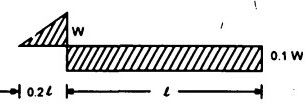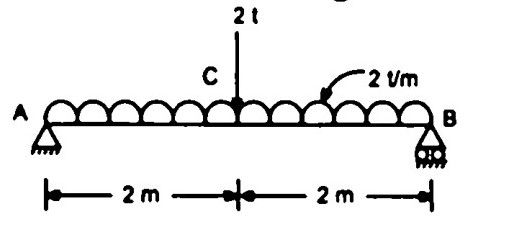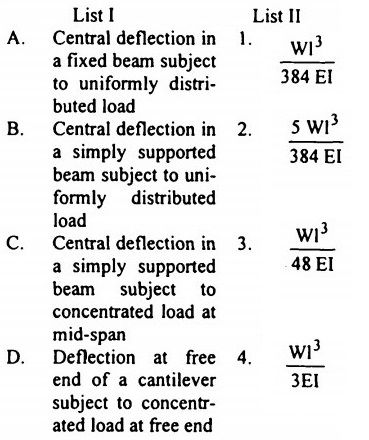top of pageSearch

# Strength of Materials(341-360)

Updated: Apr 29, 2020

341. Figure 10.82 shows a beam cantilevering out at one end. It carries a uniformly

distributed load W over the cantilever.solution342. A simply supported beam of span l is loaded (as shown in Fig. ) with a

uniformly varying load of intensity w/ unit length at 'A' to w/ unit length at 'B'The shear force at the support 4B' is given by343. A cantilever beam of span 4 m and cross sectional area 0.3 m wide and 0.4 m deep is subjected to a concentrated load of 10 kN at the free end. Neglecting self-weight, the maximum bending stress at a section 2 m from the free end will be

2500 kN/m²

344.A beam of rectangular section 100 mm x 300 mm carries certain loads such that the Bending Moment at a section A is M and at another section B it is (M+C). The distance between the sections A and B is 0.5 m and there are no external loads acting between the two sections. If the value of C is 10,000 Nm, then the

maximum shear stress is 1.0 MN/m²

345.A cantilever is subjected to a uniformly distributed load W over its whole length 'L' and a concentrated upward force W at its free end. The deflection of the free end is346.A steel rod of circular section tapers from 2 cm diameter to 1 cm diameter over a length of 50 cm. If the modulus of elasticity of the material is 2 x 10

kg/cm², then the increase in length under a pull of 3000 kg will be

(0.3/2π)cm

347. A simply supported beam AB of span 4 m carries loads as shown in Fig.The corresponding SFD and BMD for the beam will be as shown in348. For the system of springs shown in Fig ,the equivalent spring stiffness is58 kN/m

349.A cantilever beam of span L carries concentrated load 'W at the free end. If the width 'b' of the beam is constant throughout the span, then for the beam to have uniform strength, the depth 'd' at the free end should b350.

1. the material of the beam is homogeneous, isotropic and obeys Hook's law

2. plane sections of the beam remain plane after bending.

4. Young's moduli are the same for tension and compression.

351.In a simply supported beam shown in Fig. if dimensions ac and ab are

doubled, then the bending moment and the shear force at 'c' will be such thatSF is double and BM is four times

352. A rectangular beam 10 cm wide, is subjected to a maximum shear force of

50000 N, the corresponding maximum shear stress being 3 N/mm2. The depth of

the beam is 25 cm

353.354. A 4-metre long beam, simply supported at its ends, carries a point load 4W at its centre. If the slope at the ends of the beam is 1°, then the deflection at the centre of the beam will be. 23.27 mm

355. Consider the following statements about flitched beams:

1. A flitched beam has a composite section made of two or more materials joined together in such a manner that they behave as a unit piece and each material bends to the same radius of curvature.

2. The total moment of resistance of a flitched beam is equal to the sum of

the moments of resistance of individual sections.

3. Flitched beams are used when a beam of one material, if used alone, would

require quite a large cross sectional area

356.357. In order to produce a maximum shearing stress of 75 MN/m² in the material of a hollow circular shaft of 25 cm outer diameter and 17.5 cm inside diameter, the

torque that should be applied to the shaft is 174.9 kN.m

358. Strain energy in a member is a function of strains as well as stresses

359

A. Ratio of lateral strain to linear strain - Poisson's ratio B. Ratio of stress to strain - Young's modulus C. Ratio of extension to original length - Strain D. Ratio of axial pull to area of section - Tensile stress

360.Which one of the following represents the correct shear force diagram of the simply supported beam shown in Fig